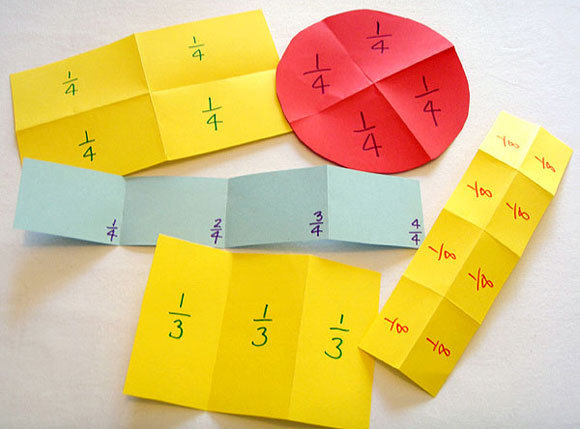Home > Fractions > Activities > Paper folding

# Paper folding

Students explore folding paper shapes into equal parts and labelling the parts with written fractions.

Select the shapes to be used according to the type of fraction being studied. Rectangles and thin strips are suitable for all fractions. Circles and triangles are less versatile.

Ensure students understand whether they are dividing into equal areas or equal lengths.

Constantly emphasise the importance of the parts being equal in size. Ask students to explain how they know they have actually represented the particular fraction correctly.Folded and labelled paper shapes.

For shapes and fractions that are too difficult to divide by folding (e.g. folding a circle into thirds), students can estimate where to draw the dividing lines. They can then cut out the parts, and directly compare the areas.

Use questioning and discussion to prompt students to realise that:

• the same fraction can be represented in a variety of ways, often by dividing the same shape in different ways
• the same shape can be used to model a variety of different fractions.